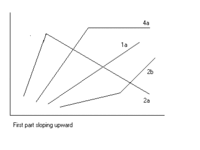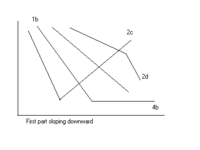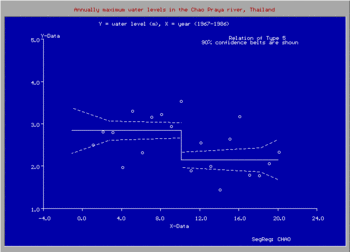# Segmented regression

﻿
Segmented regression

Segmented regression is a method in regression analysis in which the independent variable is partitioned into intervals and a separate line segment is fit to each interval. Segmented or piecewise regression analysis can also be performed on multivariate data by partitioning the various independent variables. Segmented regression is useful when the independent variables, clustered into different groups, exhibit different relationships between the variables in these regions. The boundaries between the segments are breakpoints.Segmented linear regression

Segmented linear regression is segmented regression whereby the relations in the intervals are obtained by linear regression.

## Segmented linear regression, two segments1st limb horizontal1st limb sloping up1st limb sloping down

Segmented linear regression with two segments separated by a breakpoint can be useful to quantify an abrupt change of the response function (Yr) of a varying influential factor (x). The breakpoint can be interpreted as a critical, safe , or threshold value beyond or below which (un)desired effects occur.

The breakpoint can be important in decision making 

A segmented regression analysis is based on the presence of a set of ( y , x ) data, in which y is the dependent variable and x the independent variable.

The least squares method applied separately to each segment, by which the two regression lines are made to fit the data set as closely as possible while minimizing the sum of squares of the differences (SSD) between observed (y) and calculated (Yr) values of the dependent variable, results in the following two equations:

• Yr = A1.x + K1     for x < BP (breakpoint)
• Yr = A2.x + K2     for x > BP (breakpoint)

where:

Yr is the expected (predicted) value of y for a certain value of x;
A1 and A2 are regression coefficients (indicating the slope of the line segments);
K1 and K2 are regression constants (indicating the intercept at the y-axis).

The data may show many types or trends, see the figures.

The method also yields two correlation coefficients (R):

• (R1)2 = 1 − sum { (y − Yr)2 } / sum { (y − Ya1)2 }     for x < BP (breakpoint)
• (R2)2 = 1 − sum { (y − Yr)2 } / sum { (y − Ya2)2 }     for x > BP (breakpoint)

where:

sum { (y − Yr)2 } is the minimimized SSD per segment;
Ya1 and Ya2 are the average values of y in the respective segments.

In the determination of the most suitable trend, statistical tests must be performed to ensure that this trend is reliable (significant).

When no significant breakpoint can be detected, one must fall back on a regression without breakpoint.

## Example

For the blue figure at the top of the page that gives the relation between yield of mustard (Yr = Ym , t/ha) and soil salinity (x = Ss , expressed as electric conductivity of the soil solution EC in dS/m) it is found that:

BP = 4.93 , A1 = 0 , K1 = 1.74 , A2 = −0.129 , K2 = 2.38 , (R1)2 = 0.0035 (insignificant) , (R2)2 = 0.395 (significant) and:

• Ym = 1.74 t/ha                        for Ss < 4.93 (breakpoint)
• Ym = −0.129 Ss + 2.38 t/ha     for Ss > 4.93 (breakpoint)

indicating that soil salinities < 4.93 dS/m are safe and soil salinities > 4.93 dS/m reduce the yield @ 0.129 t/ha per unit increase of soil salinity.

The figure also shows confidence intervals and uncertainty as eleborated hereunder.

## Test proceduresExample time series, type 5

The following statistical tests are used to determine the type of trend:

1. significance of the breakpoint (BP) by expressing BP as a function of regression coefficients A1 and A2 and the means Y1 and Y2 of the (y) data and the means X1 and X2 of the x data (left and an right of BP), using the laws of propagation of errors in additions and multiplications to compute the standard error (SE) of BP, and applying Student's t-test
2. significance of A1 and A2 applying Student's t-distribution and the standard error SE of A1 and A2
3. significance of the difference of A1 and A2 applying Student's t-distribution using the SE of the difference.
4. significance of the difference of Y1 and Y2 applying Student's t-distribution using the SE of the difference.

In addition, use is made of the correlation coefficient of all data (Ra) , the coefficient of determination or coefficient of explanation , confidence intervals of the regression functions , and Anova analysis.

The coefficient of determination for all data (Cd) , that is to be maximised under the conditions set by the significance tests, is found from:

• Cd = 1 − sum { (y − Yr)2 } / sum { (y − Ya)2 }

where Yr is the expected (predicted) value of y according to the former regression equations and Ya is the average of all y values.

The Cd coefficient ranges between 0 (no explanation at all) to 1 (full explanation, perfect match).
In a pure, unsegmented, linear regression, the values of Cd and Ra2 are equal. In a segmented regression, Cd needs to be significantly larger than Ra2 to justify the segmentation.

The optimal value of the breakpoint may be found such that the Cd coefficient is maximum.

Wikimedia Foundation. 2010.

### Look at other dictionaries:

• Regression analysis — In statistics, regression analysis is a collective name for techniques for the modeling and analysis of numerical data consisting of values of a dependent variable (response variable) and of one or more independent variables (explanatory… …   Wikipedia

• Linear regression — Example of simple linear regression, which has one independent variable In statistics, linear regression is an approach to modeling the relationship between a scalar variable y and one or more explanatory variables denoted X. The case of one… …   Wikipedia

• Nonlinear regression — See Michaelis Menten kinetics for details In statistics, nonlinear regression is a form of regression analysis in which observational data are modeled by a function which is a nonlinear combination of the model parameters and depends on one or… …   Wikipedia

• Robust regression — In robust statistics, robust regression is a form of regression analysis designed to circumvent some limitations of traditional parametric and non parametric methods. Regression analysis seeks to find the effect of one or more independent… …   Wikipedia

• Outline of regression analysis — In statistics, regression analysis includes any technique for learning about the relationship between one or more dependent variables Y and one or more independent variables X. The following outline is an overview and guide to the variety of… …   Wikipedia

• Multivariate adaptive regression splines — (MARS) is a form of regression analysis introduced by Jerome Friedman in 1991. It is a non parametric regression technique and can be seen as an extension of linear models that automatically models non linearities and interactions. The term… …   Wikipedia

• List of statistics topics — Please add any Wikipedia articles related to statistics that are not already on this list.The Related changes link in the margin of this page (below search) leads to a list of the most recent changes to the articles listed below. To see the most… …   Wikipedia

• Ordinary least squares — This article is about the statistical properties of unweighted linear regression analysis. For more general regression analysis, see regression analysis. For linear regression on a single variable, see simple linear regression. For the… …   Wikipedia

• Least squares — The method of least squares is a standard approach to the approximate solution of overdetermined systems, i.e., sets of equations in which there are more equations than unknowns. Least squares means that the overall solution minimizes the sum of… …   Wikipedia

• Errors and residuals in statistics — For other senses of the word residual , see Residual. In statistics and optimization, statistical errors and residuals are two closely related and easily confused measures of the deviation of a sample from its theoretical value . The error of a… …   Wikipedia